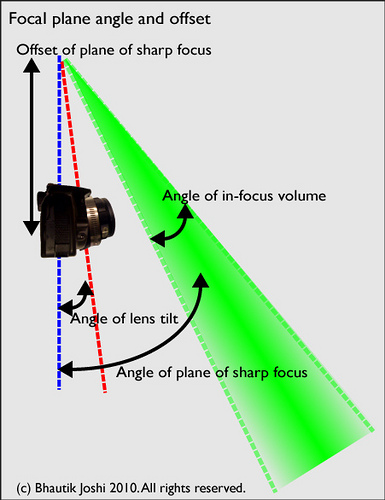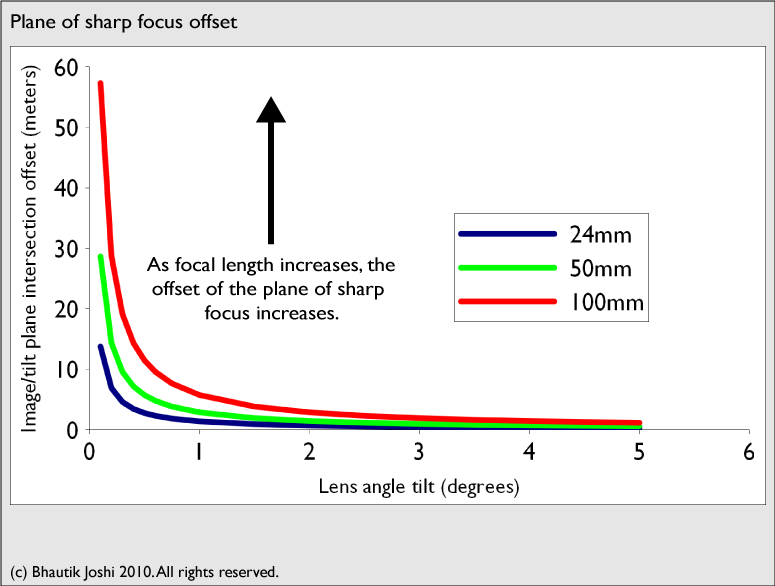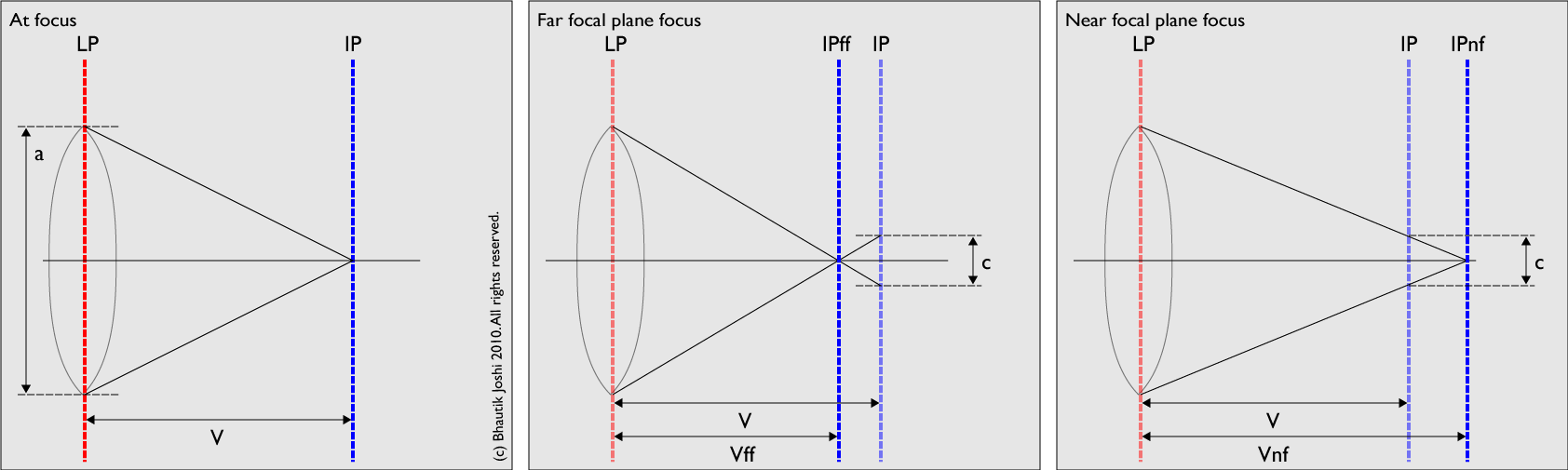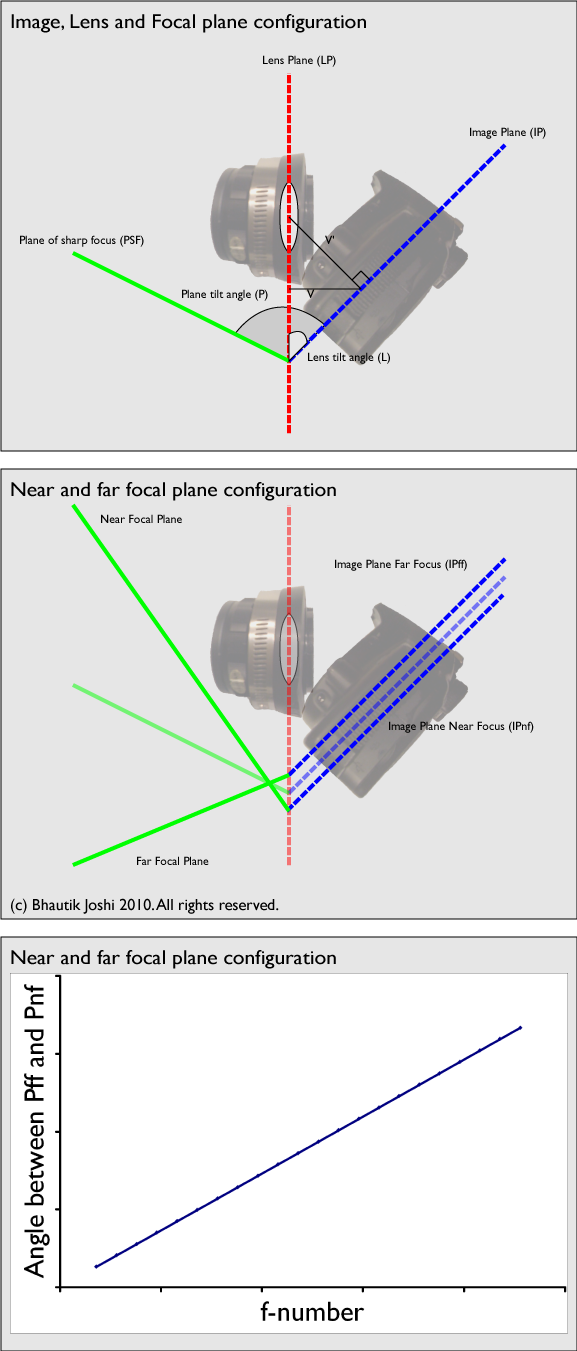# Tilt-shift, a DIY guide - part 6, Appendices

Posted on Tue 06 July 2010

## Appendix A: lens relationships

As mentioned earlier, the modern analysis of tilt-shift lenses was developed by Scheimpflug in the early part of last century. He developed a number of equations to describe what happens to the focal planes of a camera and lens combination when the lens is tilted.

The equations below make a number of assumptions to simplify the discussion, not least of which is that we assume that the lens is reduced to a thin-lens approximation for calculations. They by no means produce very accurate numbers, but given the approximate nature of the measurements in the DIY-lens building they are meant mostly just to illustrate the rough characteristics of the lens as various parameters are changed.Tilt angle analysis

When the lens plane is tilted relative to the image plane, they intersect along a line. From this line, a focal wedge is projected, bounded on one side by the near focal plane, on the other by the far focal plane and in-between by the optimal plane of sharp focus. This wedge is offset from the centre of the the image plane by an offset, J:

`J = f / sin (lensang)`

where: J = offset distance f = focal length (in mm) lensang = lens angle tilt to image plane in degreesFocal plane analysis

As shown in tilt offset analysis, as the focal length increases, the offset of the intersection also increases, decreasing as lens tilt increases. A long offset is useful when one wishes to isolate a distant object in-focus while still remaining on the ground (such as a building). This permits the user to use the focal wedge as a kind of focus-isolating flashlight that is held at a long distance from the camera.

The angle of the plane of sharp focus is governed by the equation:

`sharp focus angle = atan ((objdist/f) * sin (lensang))`

where: objdist = distance to object being focused on (in mm) f = focal length (in mm) lensang = lens angle tilt to image plane in degrees

From the above equation, it can be seen that that the sharp focus angle is inversely proportional to the focal length.Focal plane limits

Proving that the f-stop affects the angle of the in-focus volume is a bit more involved. In the leftmost box of the above diagram, we measure the back focal distance - approximated as the distance between the lens plane (LP) and the image plane (IP) when they are parallel to each other - as V. The size of the aperture is a, in mm. It's worth noting that:

`N = f/a`

where: N = f-number (e.g. 1.8, 4, 5.6 etc) f = focal length (in mm) a = aperture size (sometimes known as diameter of the entrance pupil - in mm)

To find the position of the near and far focal planes, you need to know how much blurriness you image can tolerate before the object is out of focus. This is defined as the maximum size point source of light then can become on the image plane, and is known as c, the circle of confusion. It is usually quite small (e.g. in the Canon 7D is is 0.019mm).

In the middle box of the above digram, we can see that if the the light from a point source moved away from the camera, it focuses at a point just in front of the image plane and creates a circle of size c on a sensor. We'll nominate this back focal distance for the far focal plane as as Vff. Similarly, for a tolerably out of focus object near to the camera (i.e. the near focal plane) in the rightmost box of the above digram, we'll nominate the back focal distance for the near focal plane as Vnf.

Using similar triangles, we can state that:

`(V - Vff)/c = Vff/a`

Which rearranges to:

`Vff = v/((c/a) + 1)`

Similarly:

`(Vnp - v)/c = Vnp/a`

Which rearranges to:

`Vnp = v/((c/a) - 1)`Focal plane configuration

In the top box of the above diagram, you can see the standard configuration for a tilted lens with the lens plane (LP) titled to the image plane (IP), and the plane of sharp focus emerging from the intersection of the two. We nominate the perpendicular distance between the IP and the LP relative to IP as V', and the perpendicular distance from the LP to IP as V. Given a lens tilt angle of L, we can write:

`V=V'cos(L)`

Using an alternative form of Scheimpflug's equation, we can say that:

`P = atan (V'sin(L) / (V'cos(L) - f)`

The near and far tilted focal planes can be determined by substituting V' with Vff' and Vnp, as shown in the lower box of the above diagram; i.e.

`Pff = atan (Vff'sin(L) / (Vff'cos(L) - f)`

`Pnf = atan (Vnf'sin(L) / (Vnf'cos(L) - f)`

For any given arbitrary focal length and lens tilt angle, there is an almost linear relationship between f-number and the angle between Pff and Pnf, as plotted in the bottom of the above diagram.

## Appendix B: Commercially available options

There are many commercial options available for tilt-shift lenses. Unlike the current crop of DIY options, many of these allow for precise and measured control of lens movements, which can be essential for work such as perspective correction for architectural photography. I'll briefly go over a small selection of commercially available options here.

### View camera

View format cameras describe a class of camera where typically an articulated lens plate connected to a large film/sensor plate (usually bigger than 9x12cm (4x5 inches)) by bellows. The lens is designed to cast a large imaging circle and can be adjusted with a large range of motions. This is a classic camera type, used by many pioneering photographers (such as Ansel Adams), though it's versatility for perspective correction and depth of field adjustment didn't come until later. An excellent writeup on view camera movements can be found on the wikipedia page, and an excellent writeup on the geometry, optics and mathematics of lens movements (with special regard to view cameras) by Merklinger can be found here.

### Lensbabies

The lensbaby is an inexpensive, easy-to-use option if you'd like to start out experimenting with selective focus shooting but don't want to build your own. There are several versions available, each performing a number of different and clever tricks with manipulating the optical path in the lens; an excellent writeup on the lenses can be found at dansdata, here. John Swierzbin points out that 'off-axis aberrations such as coma and field curvature cause the distinctive "Lensbaby Look" in which the image is increasingly blurred as you move away from the on-axis sweet spot. Although focal plane tilt is also present, this effect is generally drowned out by the radial blur from the off-axis aberrations.'

### Actual tilt-shift lenses

Both Canon and Nikon offer precisely engineered tilt-shift lenses for use with their 35mm cameras. These often tend to be quite wide and fast, and are an excellent choice if you need reproducible, reliable results, as is often the case when you are shooting professionally. They are, however, not cheap - at the time of writing, the cheapest Canon started at ~US\$1350, and the cheapest Nikon was ~US\$2000.